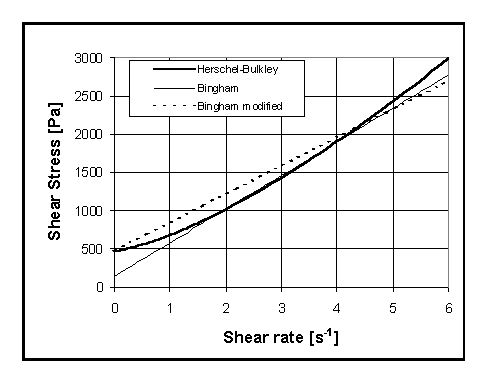# 1.5.2: Non-Newtonian Fluids

$$\newcommand{\vecs}{\overset { \rightharpoonup} {\mathbf{#1}} }$$ $$\newcommand{\vecd}{\overset{-\!-\!\rightharpoonup}{\vphantom{a}\smash {#1}}}$$$$\newcommand{\id}{\mathrm{id}}$$ $$\newcommand{\Span}{\mathrm{span}}$$ $$\newcommand{\kernel}{\mathrm{null}\,}$$ $$\newcommand{\range}{\mathrm{range}\,}$$ $$\newcommand{\RealPart}{\mathrm{Re}}$$ $$\newcommand{\ImaginaryPart}{\mathrm{Im}}$$ $$\newcommand{\Argument}{\mathrm{Arg}}$$ $$\newcommand{\norm}{\| #1 \|}$$ $$\newcommand{\inner}{\langle #1, #2 \rangle}$$ $$\newcommand{\Span}{\mathrm{span}}$$ $$\newcommand{\id}{\mathrm{id}}$$ $$\newcommand{\Span}{\mathrm{span}}$$ $$\newcommand{\kernel}{\mathrm{null}\,}$$ $$\newcommand{\range}{\mathrm{range}\,}$$ $$\newcommand{\RealPart}{\mathrm{Re}}$$ $$\newcommand{\ImaginaryPart}{\mathrm{Im}}$$ $$\newcommand{\Argument}{\mathrm{Arg}}$$ $$\newcommand{\norm}{\| #1 \|}$$ $$\newcommand{\inner}{\langle #1, #2 \rangle}$$ $$\newcommand{\Span}{\mathrm{span}}$$$$\newcommand{\AA}{\unicode[.8,0]{x212B}}$$Figure 1.7. The shear stress as a function of the sear rate.

In equation ___, the relationship between the velocity and the shear stress was assumed to be linear. Not all the materials obey this relationship. There is a large class of materials which shows a non-linear relationship with velocity for any shear stress. This class of materials can be approximated by a single polynomial term that is $$a = bx^n$$. From the physical point of view, the coefficient depends on the velocity gradient. This relationship is referred to as power relationship and it can be written as $\tau = \overbrace{K (\frac{dU}{dx})^{n-1}}^{viscosity} \left(\frac{dU}{dx}\right)$ The new coefficients $$(n, K)$$ in equation ___ are constant. When $$n = 1$$ equation represent Newtonian fluid and $$K$$ becomes the familiar $$\mu$$. The viscosity coefficient is always positive. When $$n$$ is above one, the liquid is dilettante. When $$n$$ is below one, the fluid is pseudoplastic. The liquids which satisfy equation ___ are referred to as purely viscous fluids. Many fluids satisfy the above equation. Fluids that show increase in the viscosity (with increase of the shear) referred to as thixotropic and those that show decrease are called rheopectic fluids (see Figure 1.5).

Materials which behave up to a certain shear stress as a solid and above it as a liquid are referred as Bingham liquids. In the simple case, the liquid side'' is like Newtonian fluid for large shear stress. The general relationship for simple Bingham flow is $\tau_{xy} = -\mu \cdot \pm \tau_{0} \quad if \lvert\tau_{yx}\rvert > \tau_{0}$ $\frac{dU_{x}}{dy} = 0 \quad if\lvert\tau_{yx}\rvert < \tau_{0}$ There are materials that simple Bingham model does not provide adequate explanation and a more sophisticate model is required. The Newtonian part of the model has to be replaced by power liquid. For example, according to Ferraris at el concrete behaves as shown in Figure 1.7. However, for most practical purposes, this kind of figures isn't used in regular engineering practice.

This page titled 1.5.2: Non-Newtonian Fluids is shared under a GNU Free Documentation License 1.3 license and was authored, remixed, and/or curated by via source content that was edited to the style and standards of the LibreTexts platform; a detailed edit history is available upon request.

This page titled 1.5.2: Non-Newtonian Fluids is shared under a GNU Free Documentation License 1.3 license and was authored, remixed, and/or curated by Genick Bar-Meir via source content that was edited to the style and standards of the LibreTexts platform; a detailed edit history is available upon request.